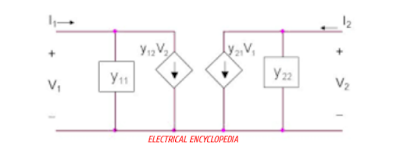# How To Find Y Parameters of Two Port Network (Examples)

0
185

## Y-PARAMETER of Two Port Network

### CASE-1 SHORT CIRCUITING THE OUTPUT PORT.

#### If the Output port of the two port network is  short circuited then output voltage is zero, i.e. V2 = 0.### CASE-2 SHORT CIRCUITING THE INPUT PORT.

####Now, equation 1 and 2 will be reduced to : I1 = Y12* V2 I2 = Y22* V2So, Y12 = I1/ V2    ( keeping V1= 0 )       Y22 = I2/ V2  ( keeping V1 = 0 )Y11 is called input driving point Admittance. Y12 is called forward transfer Admittance. Y21 is called reverse transfer Admittance Y22 is called output driving point Admittance.For a network to be symmetrical , Y11 = Y22 For a network to be reciprocal, Y12 = Y21